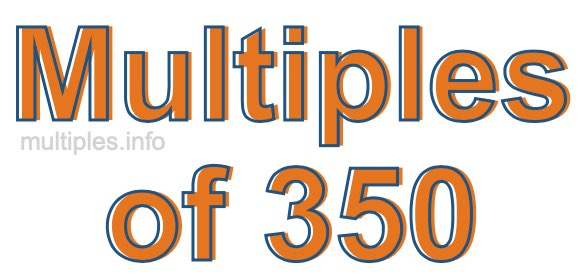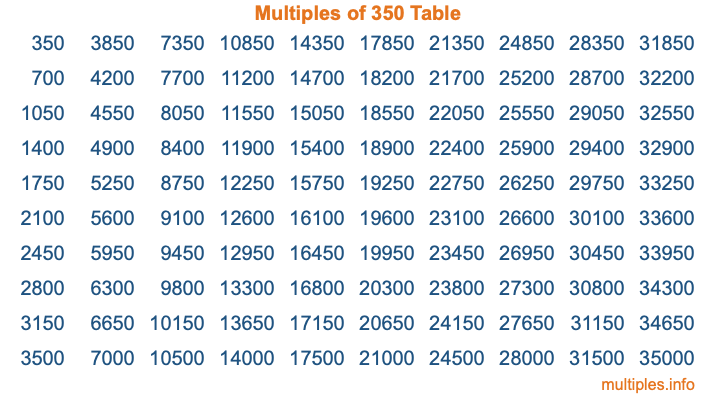Multiples of 350Welcome to the Multiples of 350 page. Here we will first teach you everything you will ever need to know about the multiples of 350, and then give you a study guide summary of everything we taught you to make sure you remember it all. Use this page to look up facts and learn information about the multiples of 350. This page will make you a multiples of three hundred fifty expert!

Definition of Multiples of 350
Multiples of 350 are all the numbers that when divided by 350 equal an integer. Each of the multiples of 350 are called a multiple. A multiple of 350 is created by multiplying 350 by an integer.

Therefore, to create a list of multiples of 350, you start with 1 multiplied by 350, then 2 multiplied by 350, then 3 multiplied by 350, and so on for as long as you want. Thus, the list of the first five multiples of 350 is 350, 700, 1050, 1400, and 1750. To see a larger list of multiples of 350, see the printable image of Multiples of 350 further down on this page. We also have a category where you can choose any nth multiple of 350.

Multiples of 350 Checker
The Multiples of 350 Checker below checks to see if any number of your choice is a multiple of 350. In other words, it checks to see if there is any number (integer) that when multiplied by 350 will equal your number. To do that, we divide your number by 350. If the the quotient is an integer, then your number is a multiple of 350.

Is  a multiple of 350?

Least Common Multiple of 350 and ...
A Least Common Multiple (LCM) is the lowest multiple that two or more numbers have in common. This is also called the smallest common multiple or lowest common multiple and is useful to know when you are adding our subtracting fractions. Enter one or more numbers below (350 is already entered) to find the LCM.

Check out our LCM Calculator if you need more details about the Least Common Multiple or if you need the LCM for different numbers for adding and subtraction fractions.

nth Multiple of 350
As we stated above, 350 is the first multiple of 350, 700 is the second multiple of 350, 1050 is the third multiple of 350, and so on. Enter a number below to find the nth multiple of 350.

th multiple of 350

Multiples of 350 vs Factors of 350
350 is a multiple of 350 and a factor of 350, but that is where the similarities end. All postive multiples of 350 are 350 or greater than 350. All positive factors of 350 are 350 or less than 350.

Below is the beginning list of multiples of 350 and the factors of 350 so you can compare:

Multiples of 350: 350, 700, 1050, 1400, 1750, etc.

Factors of 350: 1, 2, 5, 7, 10, 14, 25, 35, 50, 70, 175, 350

As you can see, the multiples of 350 are all the numbers that you can divide by 350 to get a whole number. The factors of 350, on the other hand, are all the whole numbers that you can multiply by another whole number to get 350.

It's also interesting to note that if a number (x) is a factor of 350, then 350 will also be a multiple of that number (x).

Multiples of 350 vs Divisors of 350
The divisors of 350 are all the integers that 350 can be divided by evenly. Below is a list of the divisors of 350.

Divisors of 350: 1, 2, 5, 7, 10, 14, 25, 35, 50, 70, 175, 350

The interesting thing to note here is that if you take any multiple of 350 and divide it by a divisor of 350, you will see that the quotient is an integer.

Multiples of 350 Table
Below is an image of the first 100 multiples of 350 in a table. The table is in chronological order, column by column. The first column has the first ten multiples of 350, the second column has the next ten multiples of 350, and so on.The Multiples of 350 Table is also referred to as the 350 Times Table or Times Table of 350. You are welcome to print out our table for your studies.

Negative Multiples of 350
Although not often discussed or needed in math, it is worth mentioning that you can make a list of negative multiples of 350 by multiplying 350 by -1, then by -2, then by -3, and so on, to get the following list of negative multiples of 350:

-350, -700, -1050, -1400, -1750, etc.

Multiples of 350 Summary
Below is a summary of important Multiples of 350 facts that we have discussed on this page. To retain the knowledge on this page, we recommend that you read through the summary and explain to yourself or a study partner why they hold true.

There are an infinite number of multiples of 350.

A multiple of 350 divided by 350 will equal a whole number.

350 divided by a factor of 350 equals a divisor of 350.

The nth multiple of 350 is n times 350.

The largest factor of 350 is equal to the first positive multiple of 350.

350 is a multiple of every factor of 350.

350 is a multiple of 350.

A multiple of 350 divided by a divisor of 350 equals an integer.

350 divided by a divisor of 350 equals a factor of 350.

Any integer times 350 will equal a multiple of 350.

Multiples of a Number
Here you can get the multiples of another number, all with the same attention to detail as we did for multiples of 350 on this page.

Multiples of
Multiples of 351
Did you find our page about multiples of three hundred fifty educational? Do you want more knowledge? Check out the multiples of the next number on our list!